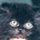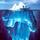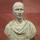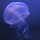## Social Question# Could someone explain this Critical angle >sin^-1 (n1/n0) in words?

Asked by Siblinings (136) February 28th, 2010

I understand what it means but I need to explain it in a power point and I’m struggling.

Observing members: 0Composing members: 0This Link gives a nice explanation. Let me know if you have any questions.

LostInParadise (25488)“Great Answer” (1) Flag as…The critical angle (where transition from refraction to total internal reflection occurs) is the angle whose sine equals the ratio of indexes of refraction.

gasman (11296)“Great Answer” (0) Flag as…The critical angle is where light is refracted at an angle such that it cannot fully pass into the less dense medium, but traverses parallel to the barrier between the two media.

FireMadeFlesh (16548)“Great Answer” (0) Flag as…This is the smallest angle to the surface normal at which total internal reflection occurs; the word definition would probably depend on the point of your powerpoint.

gagara (102)“Great Answer” (0) Flag as…